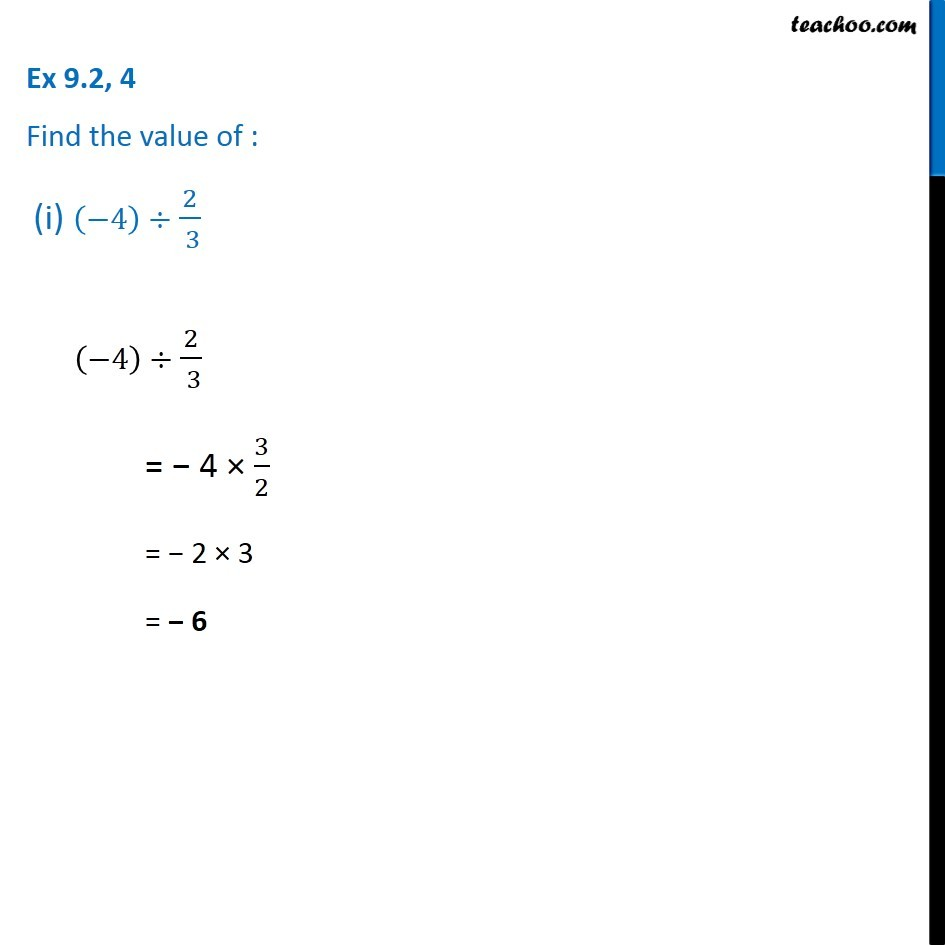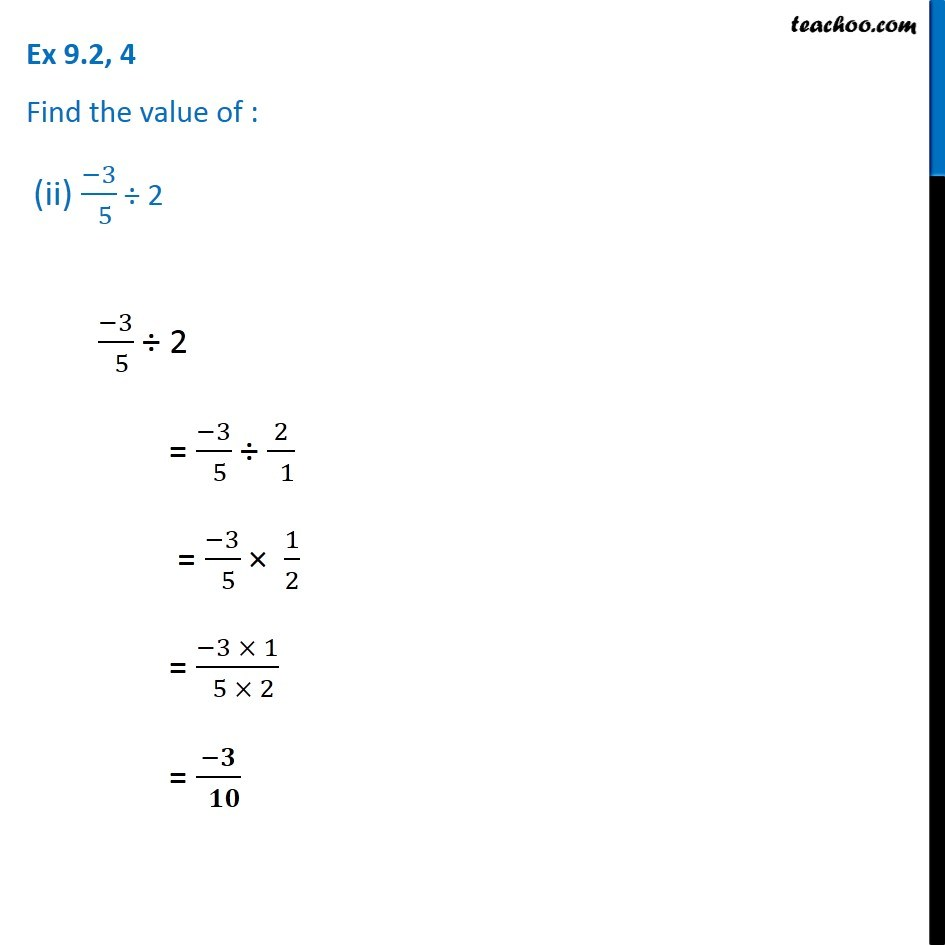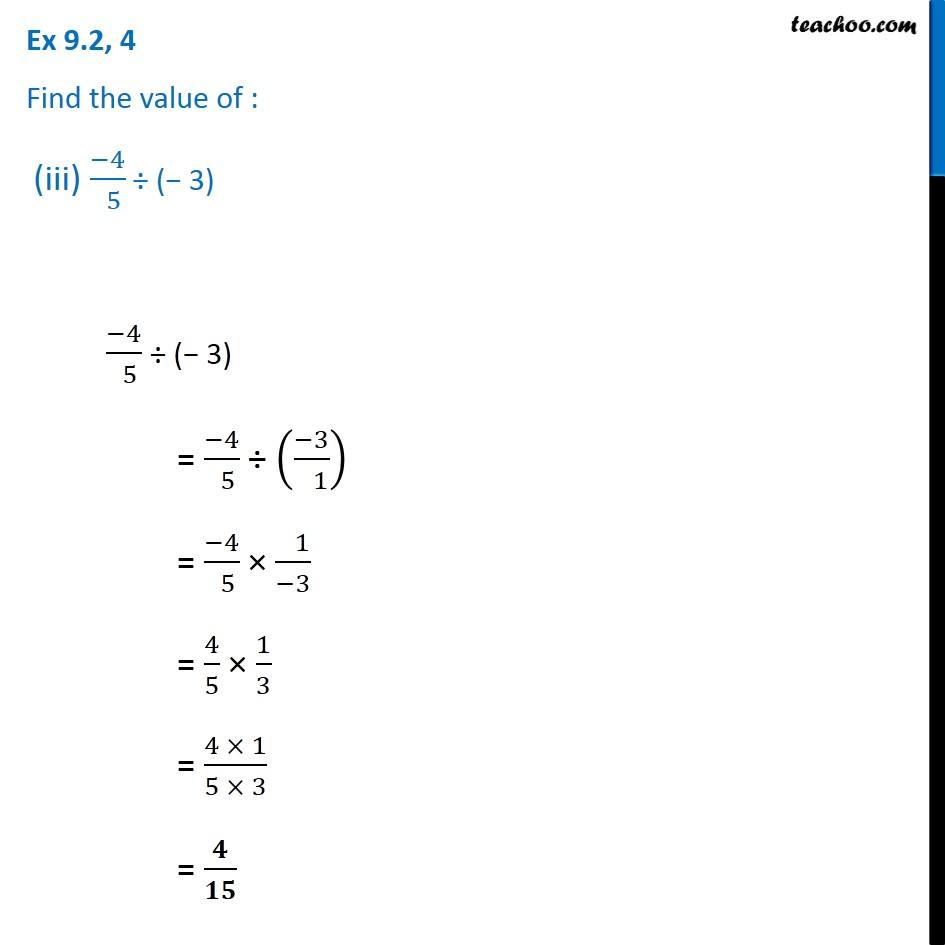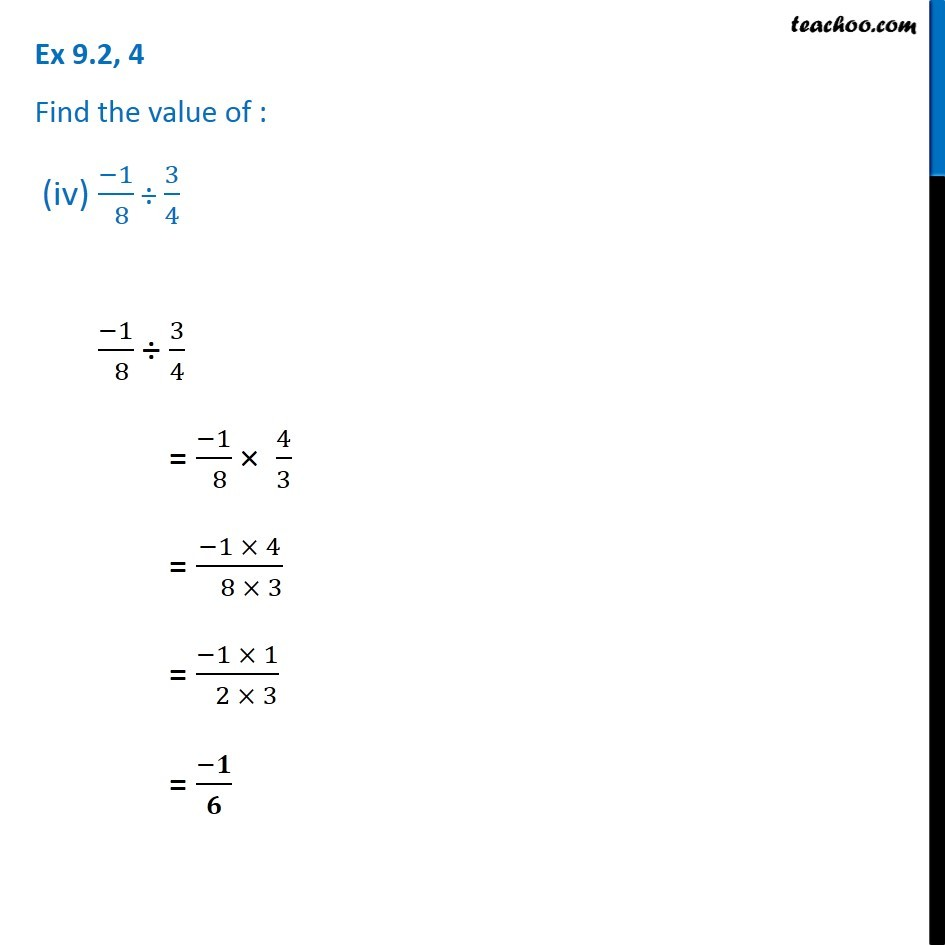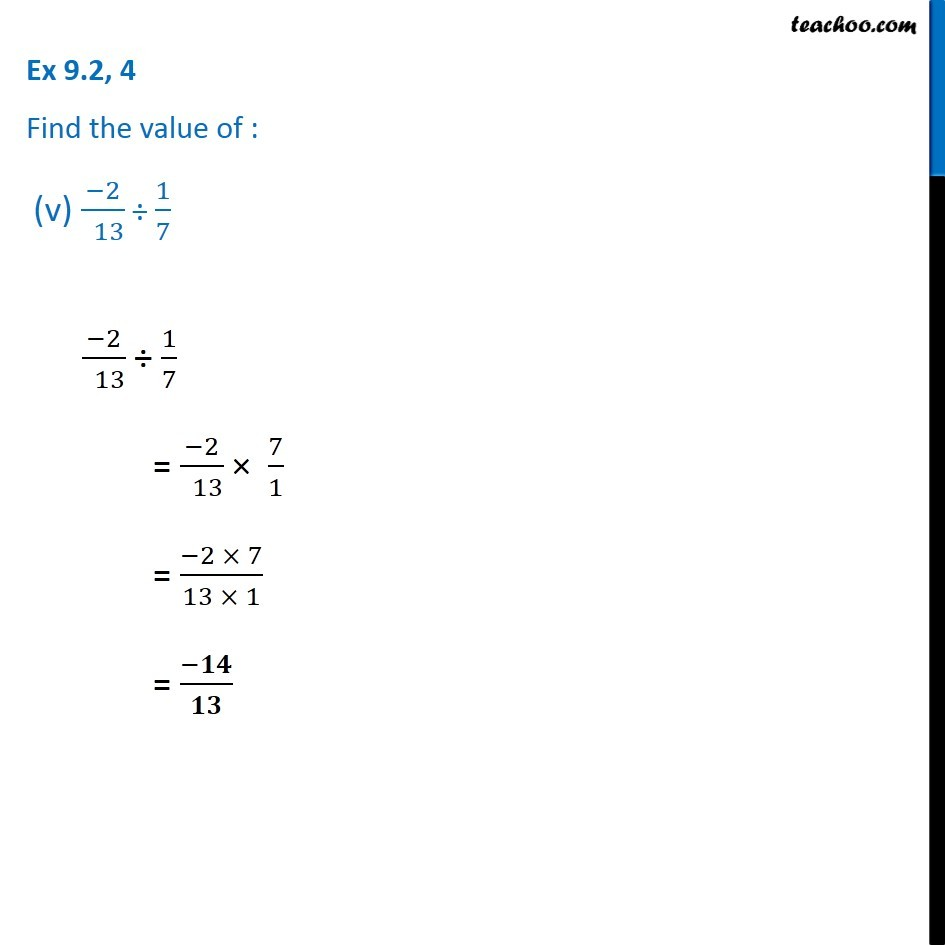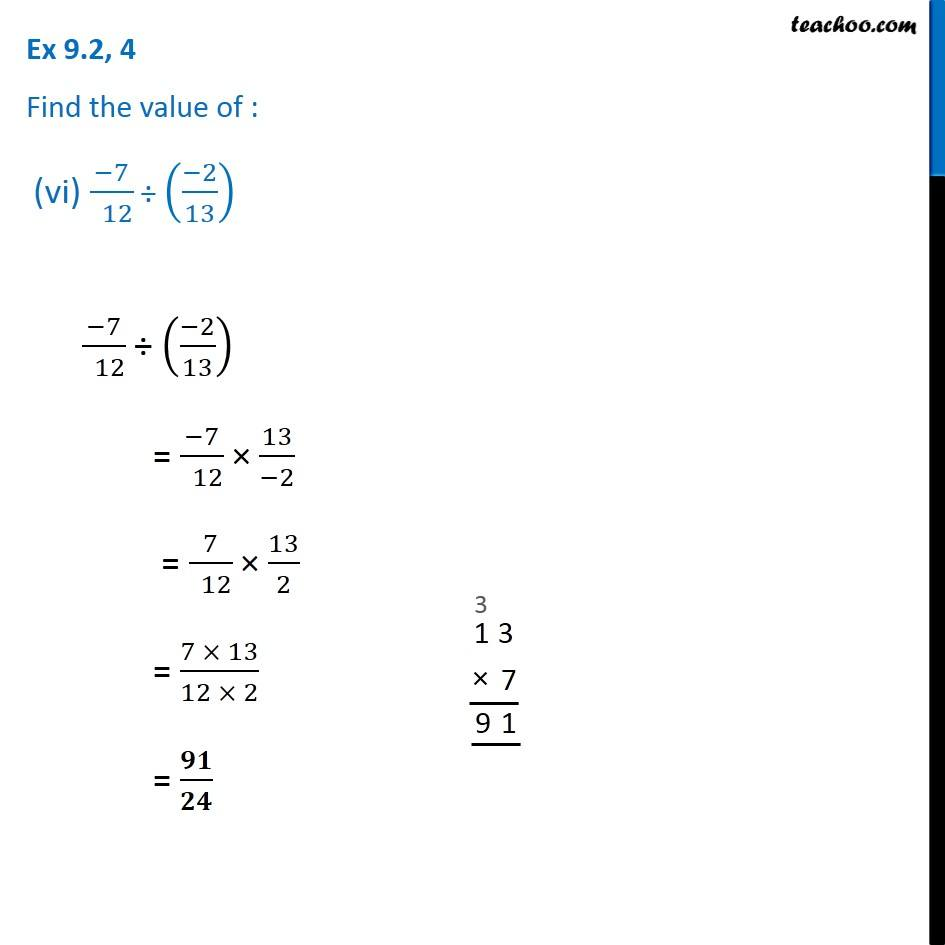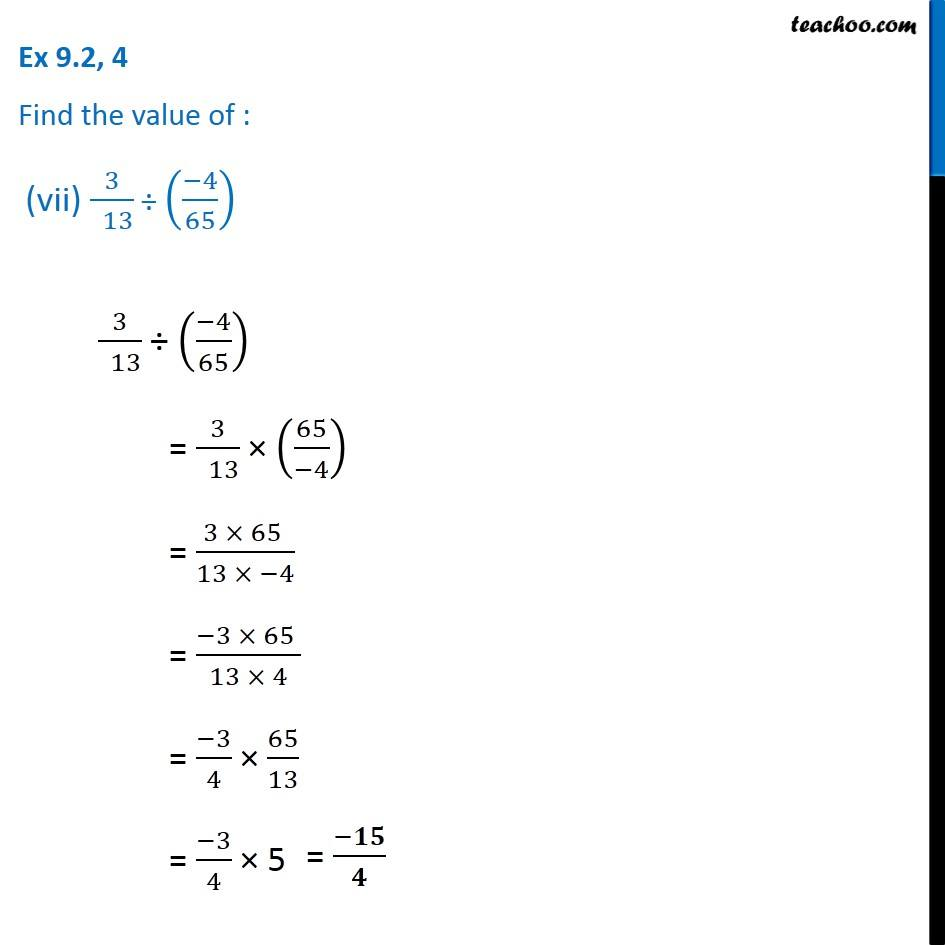1. Chapter 9 Class 7 Rational Numbers
2. Serial order wise
3. Ex 9.2

Transcript

Ex 9.2, 4 Find the value of : (i) (−4)÷ 2/( 3) (−4)÷ 2/( 3) = − 4 × 3/2 = − 2 × 3 = − 6 Ex 9.2, 4 Find the value of : (ii) (−3)/( 5) ÷ 2 (−3)/( 5) ÷ 2 = (−3)/( 5) ÷ 2/( 1) = (−3)/( 5) × 1/2 = (−3 × 1)/( 5 × 2) = (−𝟑)/( 𝟏𝟎) Ex 9.2, 4 Find the value of : (iii) (−4)/( 5) ÷ (− 3) (−4)/( 5) ÷ (− 3) = (−4)/( 5) ÷ ((−3)/( 1)) = (−4)/( 5) × ( 1)/(−3) = 4/5 × 1/3 = (4 × 1)/(5 × 3) = 𝟒/𝟏𝟓 Ex 9.2, 4 Find the value of : (iv) (−1)/( 8) ÷ 3/4 (−1)/( 8) ÷ 3/4 = (−1)/( 8) × 4/3 = (−1 × 4)/( 8 × 3) = (−1 × 1)/( 2 × 3) = (−𝟏)/𝟔 Ex 9.2, 4 Find the value of : (v) (−2)/( 13) ÷ 1/7 (−2)/( 13) ÷ 1/7 = (−2)/( 13) × 7/1 = (−2 × 7)/(13 × 1) = (−𝟏𝟒)/𝟏𝟑 Ex 9.2, 4 Find the value of : (vi) (−7)/( 12) ÷ ((−2)/13) (−7)/( 12) ÷ ((−2)/13) = (−7)/( 12) × 13/(−2) = 7/( 12) × 13/2 = (7 × 13)/(12 × 2) = 𝟗𝟏/𝟐𝟒 Ex 9.2, 4 Find the value of : (vii) 3/( 13) ÷ ((−4)/65) 3/( 13) ÷ ((−4)/65) = 3/( 13) × (65/(−4)) = (3 × 65 )/(13 × −4) = (−3 × 65 )/(13 × 4) = (−3)/4 × 65/13 = (−3)/4 × 5 = (−𝟏𝟓)/𝟒

Ex 9.2